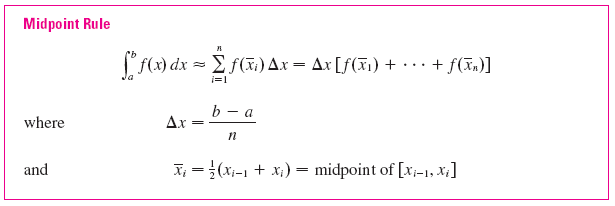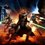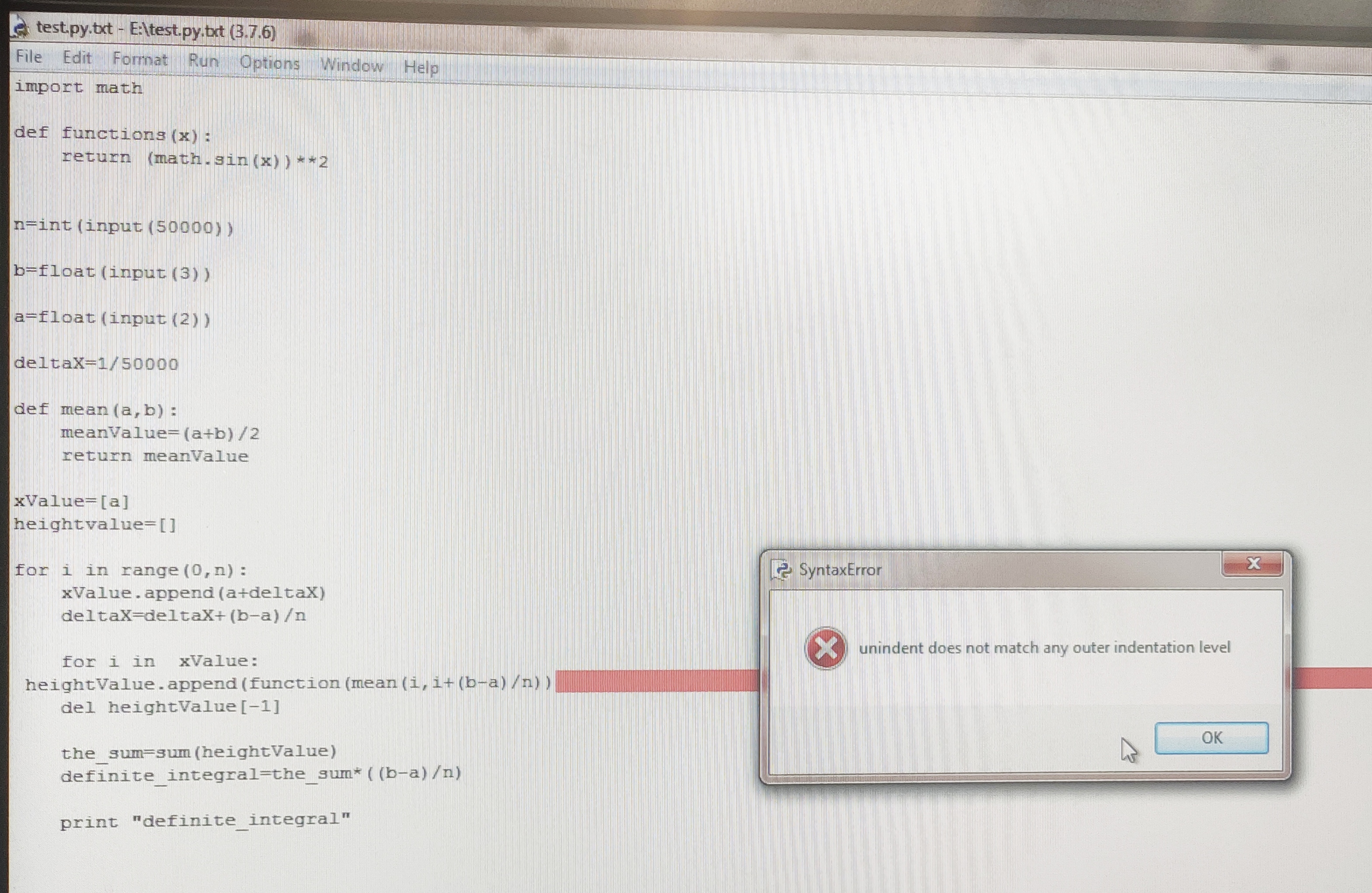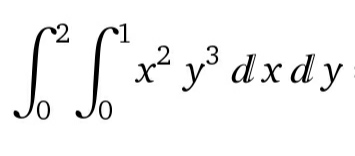# Midpoint Rule Numerical integration using python

I created a program to evaluate definite integrals using the Midpoint Rule.

Firstly, the midpoint rule states that:Since evaluating definite integrals using the midpoint rule is a repetitious method, it would be best if a program would do it. Increasing the value of n would increase the integral's accuracy, but it would be more difficult to do by hand.

Here is my python code for the definite integral of $\sin^2(x)$. You can change the function in the code to integrate any function you want. Increasing the number of midpoints increases accuracy.

Here's the code:

  1 2 3 4 5 6 7 8 9 10 11 12 13 14 15 16 17 18 19 20 21 22 23 24 25 26 27 28 29 30 31 32 33 34 35 36 37 import math def function(x): return (math.sin(x))**2 n=int(input('How many midpoints would you like to have? How much accuracy would you like to have? Enter an integer value from 1 to 50000, 50000 being the most precise (you could be more accurate, but what is the point because 50000 gives you more than 10 decimal places accuracy) and 1 being the least precise: ')) b=float(input('what top value of integration would you like to have? Enter: ')) a=float(input('what bottom value of integration would you like to have?Enter: ')) deltaX=(b-a)/n def mean(a,b): meanValue=(a+b)/2 return meanValue xValue=[a] heightValue=[] for i in range(0,n): xValue.append(a+deltaX) deltaX=deltaX+(b-a)/n for i in xValue: heightValue.append(function(mean(i,i+(b-a)/n))) del heightValue[-1] the_sum=sum(heightValue) definite_integral=the_sum*((b-a)/n) print('Definite integral evaluated by midpoint rule: '+str(definite_integral))Note by Krishna Karthik
1 year, 5 months ago

This discussion board is a place to discuss our Daily Challenges and the math and science related to those challenges. Explanations are more than just a solution — they should explain the steps and thinking strategies that you used to obtain the solution. Comments should further the discussion of math and science.

When posting on Brilliant:

• Use the emojis to react to an explanation, whether you're congratulating a job well done , or just really confused .
• Ask specific questions about the challenge or the steps in somebody's explanation. Well-posed questions can add a lot to the discussion, but posting "I don't understand!" doesn't help anyone.
• Try to contribute something new to the discussion, whether it is an extension, generalization or other idea related to the challenge.

MarkdownAppears as
*italics* or _italics_ italics
**bold** or __bold__ bold
- bulleted- list
• bulleted
• list
1. numbered2. list
1. numbered
2. list
Note: you must add a full line of space before and after lists for them to show up correctly
paragraph 1paragraph 2

paragraph 1

paragraph 2

[example link](https://brilliant.org)example link
> This is a quote
This is a quote
    # I indented these lines
# 4 spaces, and now they show
# up as a code block.

print "hello world"
# I indented these lines
# 4 spaces, and now they show
# up as a code block.

print "hello world"
MathAppears as
Remember to wrap math in $$ ... $$ or $ ... $ to ensure proper formatting.
2 \times 3 $2 \times 3$
2^{34} $2^{34}$
a_{i-1} $a_{i-1}$
\frac{2}{3} $\frac{2}{3}$
\sqrt{2} $\sqrt{2}$
\sum_{i=1}^3 $\sum_{i=1}^3$
\sin \theta $\sin \theta$
\boxed{123} $\boxed{123}$

Sort by:

@Krishna Karthik Thanks for this note. Bro can you please share a photo of the code of definite integral of $\sin^{2} x$.

- 1 week, 4 days ago

Got it man. I'll do it right now

- 1 week, 4 days ago

@Krishna Karthik Bro can we solve differential equations in python.
Like this way you have posted the midpoint rule can you also post the Simpson $1/3$ and $3/8$ rule.
Or can you give me some article where these rules are nicely illustrated.

- 1 week, 4 days ago

We can definitely solve ODEs in Python. I'll post and article as well as some stuff clarifying the theory behind it.

- 1 week, 4 days ago

@Krishna Karthik bro i am recently evaluating $\int_{2}^{3} \sin^{2} x dx$
here is my code but not getting answer

  1 2 3 4 5 6 7 8 9 10 11 12 13 14 15 16 17 18 19 20 21 22 23 24 25 26 27 28 29 30 31 32 33 print"import math def functions(x): return (math.sin(x))**2 n=int(input(50000)) b=float(input(3)) a=float(input(2)) deltaX=1/50000 def mean(a,b): meanValue=(a+b)/2 return meanValue xValue=[a] heightvalue=[] for i in range(0,n): xValue.append(a+deltaX) deltaX=deltaX+(b-a)/n for i in xValue: heightValue.append(function(mean(i,i+(b-a)/n)) del heightValue[-1] the_sum=sum(heightValue) definite_integral=the_sum*((b-a)/n) print " 

- 1 week, 4 days ago

Oh, nevermind. I'll have a look.

- 1 week, 4 days ago

@Krishna Karthik at last what we have to write after print in mid point theorem to compute the answer bro.

- 1 week, 4 days ago

Print the value of the definite integral stored by the variable "definite_integral".

- 1 week, 4 days ago

I got 0.38 approximately. The values converge well.

- 1 week, 4 days ago

And you can't put the input into the "input section". That's not how the code works. Run the code, and it will ask you to input.

- 1 week, 4 days ago

@Krishna Karthik it is showing this- 1 week, 4 days ago

Ok, you need to indent the "heightValue.append" line. It doesn't match the indentation for the forloop.

- 1 week, 4 days ago

- 1 week, 4 days ago

Sure. No problem ;)

- 1 week, 4 days ago

Ok, ill show:

  1 2 3 4 5 6 7 8 9 10 11 12 13 14 15 16 17 18 19 20 21 22 23 24 25 26 27 28 29 30 31 32 33 34 35 36 37 import math def function(x): return (math.sin(x))**2 n=int(input('How many midpoints would you like to have? How much accuracy would you like to have? Enter an integer value from 1 to 50000, 50000 being the most precise (you could be more accurate, but what is the point because 50000 gives you more than 10 decimal places accuracy) and 1 being the least precise: ')) b=float(input('what top value of integration would you like to have? Enter: ')) a=float(input('what bottom value of integration would you like to have?Enter: ')) deltaX=(b-a)/n def mean(a,b): meanValue=(a+b)/2 return meanValue xValue=[a] heightValue=[] for i in range(0,n): xValue.append(a+deltaX) deltaX=deltaX+(b-a)/n for i in xValue: heightValue.append(function(mean(i,i+(b-a)/n))) del heightValue[-1] the_sum=sum(heightValue) definite_integral=the_sum*((b-a)/n) print('Definite integral evaluated by midpoint rule: '+str(definite_integral)) 

- 1 week, 4 days ago

@Krishna Karthik bro it is the same as above .
i want the code of that particular question

- 1 week, 4 days ago

Ok, I'll modify it and show you.

- 1 week, 4 days ago

The one above is fine, but the problem is in your indentation levels.

- 1 week, 4 days ago

Enter the n, a , b values like this and it'll work:

  1 2 3 4 5 6 7 8 9 10 11 12 13 14 15 16 17 18 19 20 21 22 23 24 25 26 27 28 29 30 31 32 33 34 35 36 37 import math def function(x): return (math.sin(x))**2 n=2500 b=3 a=2 deltaX=(b-a)/n def mean(a,b): meanValue=(a+b)/2 return meanValue xValue=[a] heightValue=[] for i in range(0,n): xValue.append(a+deltaX) deltaX=deltaX+(b-a)/n for i in xValue: heightValue.append(function(mean(i,i+(b-a)/n))) del heightValue[-1] the_sum=sum(heightValue) definite_integral=the_sum*((b-a)/n) print('Definite integral evaluated by midpoint rule: '+str(definite_integral)) 

- 1 week, 4 days ago

Your indentation is incorrect, that can alter the code completely.

- 1 week, 4 days ago

Copy the code exactly as it is, with the exact same spacing.

- 1 week, 4 days ago

Copy and paste the code above without modifying it, and just run it. It'll get you to enter the values into the console. I'll share a photo with you.

- 1 week, 4 days ago

See, you have to run the code, and it will get you to enter the values. Don't modify the code yourself unless you are willing to change the function you want to integrate. If you run the code for the integral above, it will come with a menu like this:

How many midpoints would you like to have? How much accuracy would you like to have? Enter an integer value from 1 to 50000, 50000 being the most precise (you could be more accurate, but what is the point because 50000 gives you more than 10 decimal places accuracy) and 1 being the least precise: 10000 what top value of integration would you like to have? Enter: 3 what bottom value of integration would you like to have?Enter: 2 Definite integral evaluated by midpoint rule: 0.3806532505238656

- 1 week, 4 days ago

I've posted a picture of the code in a note above.

- 1 week, 4 days ago

@Krishna Karthik guide me to evaluate this using explicit euler- 1 week, 4 days ago

@Krishna Karthik bro please solve the above double integral using midpoint rule.
i am waiting

- 1 week, 3 days ago

I have posted it. Let me know if I can be of further help.

- 1 week, 2 days ago